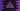# Introduction to for loop in C### For loop in C#:

For loop is used to execute a code block for a given number of times. For loop is available in almost all programming languages. C# for loop works in a similar manner.

In this post, we will learn how to use a for loop in C# with examples.

### Syntax of for loop:

Below is the syntax of for loop:

``````for (initializer; condition; iterator){
// code
}``````

There are three important parts in a for loop, separated by a semicolon. All parts are optional.

#### initializer:

Initializer section is used to initialize a variable. This variable is used as the counter variable for the for loop. This is a local variable and we can’t access it outside of the for loop.

Initializer can be:

• An assignment statement
• A method invocation
• Prefix/postfix increment or decrement expression e.g. i++, i—, ++i, —i.
• Object creation.
• await expression.

We can also have more than one among the above list. We need to separate them by comma.

#### condition:

The condition should be a boolean expression. Before each iteration, this condition is evaluated. It it returns true or it the condition is present, it executes the next iteration. Else, the loop is exited.

#### iterator:

This section runs after each iteration of the loop. It can be any of the following:

• An assignment statement
• A method invocation
• Prefix/postfix increment or decrement expression e.g. i++, i—, ++i, —i.
• Object creation.
• await expression.

### Example of for loop:

Let’s write one simple for loop:

``````using System;

public class Program
{
public static void Main()
{
for(int i = 0; i<5 ; i++){
Console.WriteLine("i = {0}",i);
}
}
}``````

Here,

• In initializer, we are initializing the integer variable i and assigning it 0.
• The condition checks if the value of i is less than 5 or not.
• The iterator increments the value of i by 1.

If you run this program, it will print the below output:

``````i = 0
i = 1
i = 2
i = 3
i = 4``````

You can see that the value i is changed on each iteration of the loop.

### You don’t need the curly braces for single line code block:

The curly braces are not required always. For example, if you have only one line in the inner code block, these are not required.

The above program has only one line in the code block. We can also write it as like below:

``````public class Program
{
public static void Main()
{
for(int i = 0; i < 5 ; i++)
Console.WriteLine("i = {0}",i);
}
}``````

### Nested for loops:

We can have for loops one inside another. This is called nested for loops. Let’s try with an example:

``````public class Program
{
public static void Main()
{
for(int i = 0; i < 3 ; i++){
for(int j = 0; j < 2 ; j++){
Console.WriteLine("i = {0}, j = {1}",i,j);
}
}
}
}``````

Here, we have two for loops, one inside another.

• The outer loop runs for i and the inner loop runs for j.
• i runs from 0 to 2 and j runs from 0 to 1 for each value of i.

If you run this program, it will print the below output:

``````i = 0, j = 0
i = 0, j = 1
i = 1, j = 0
i = 1, j = 1
i = 2, j = 0
i = 2, j = 1``````

### Infinite for loop:

An infinite for loop will run for an infinite amount of time. To create an infinite for loop, we need to create the for loop without adding any values to the initializer, condition and iterator.

Below is an infinite for loop:

``````public class Program
{
public static void Main()
{
for(;;)
Console.Write("Hello..");
}
}``````

If you run it, it will keep printing the Hello.. string infinitely. The output will be…

``Hello..Hello..Hello..Hello..Hello..Hello..Hello..Hello..Hello........``

### Running a for loop in reverse:

We can run a for loop in reverse order easily by changing the iterator section. For example,

``````public class Program
{
public static void Main()
{
for(int i = 5; i > 0 ; i--)
Console.WriteLine("i = {0}",i);
}
}``````

This program will run from i = 5 to i = 1. On each step, it is decrementing the value of i by 1. If you run this program, it will print the below output: